# Capacitors in Parallel

• Capacitors store charge on their plates

• Capacitors in parallel can be replaced with an equivalent capacitor

•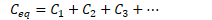# Capacitors in Series

• Charge on capacitors must be the same

• Capacitors in series replaced with an equivalent capacitor

•# RC Circuits

• RC Circuits are circuits comprised of a source of potential difference, a resistor network, and one or more capacitors

• We will look at RC circuits from the steady-state perspective

• What happens when first turned on

• What happens after a "long" time has elapsed

• Key to understanding RC Circuit Performance

• Uncharged capacitors act like wires

• Charged capacitors act like opens

# Charging an RC Circuit# Discharging an RC Circuit# The Time Constant

•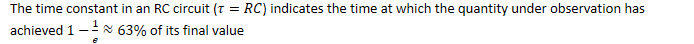•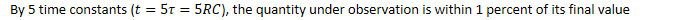# Example 1: RC Analysis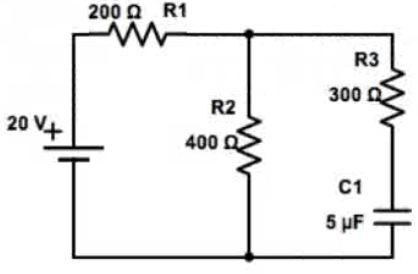• What is the current through R2 when the circuit is first connected?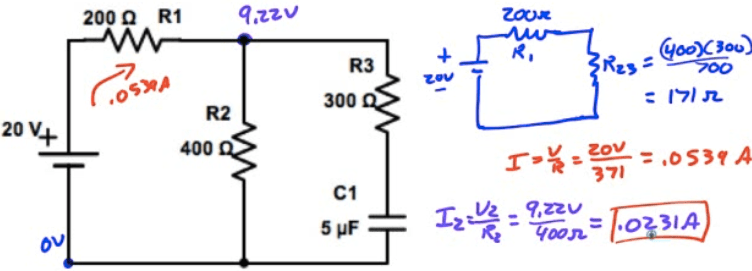• What is the current through R2 a long time after the circuit has been connected?# Example 2: More RC Analysis• What is the current through R3 when the circuit is first connected?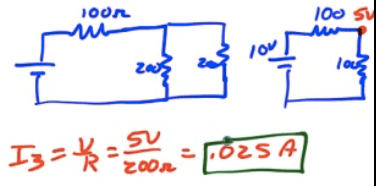• What is the current through R2 a long time after the circuit has been connected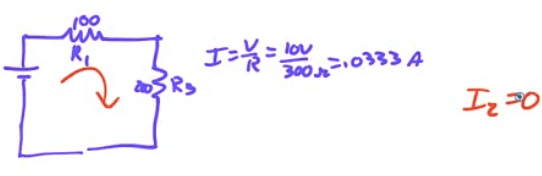# Example 3: Equivalent Capacitance

• What is the equivalent capacitance of the capacitor network shown below?# Example 4: More Equivalent Capacitance

• What is the equivalent capacitance of the capacitor network shown below?# 2010 Free Response Question 2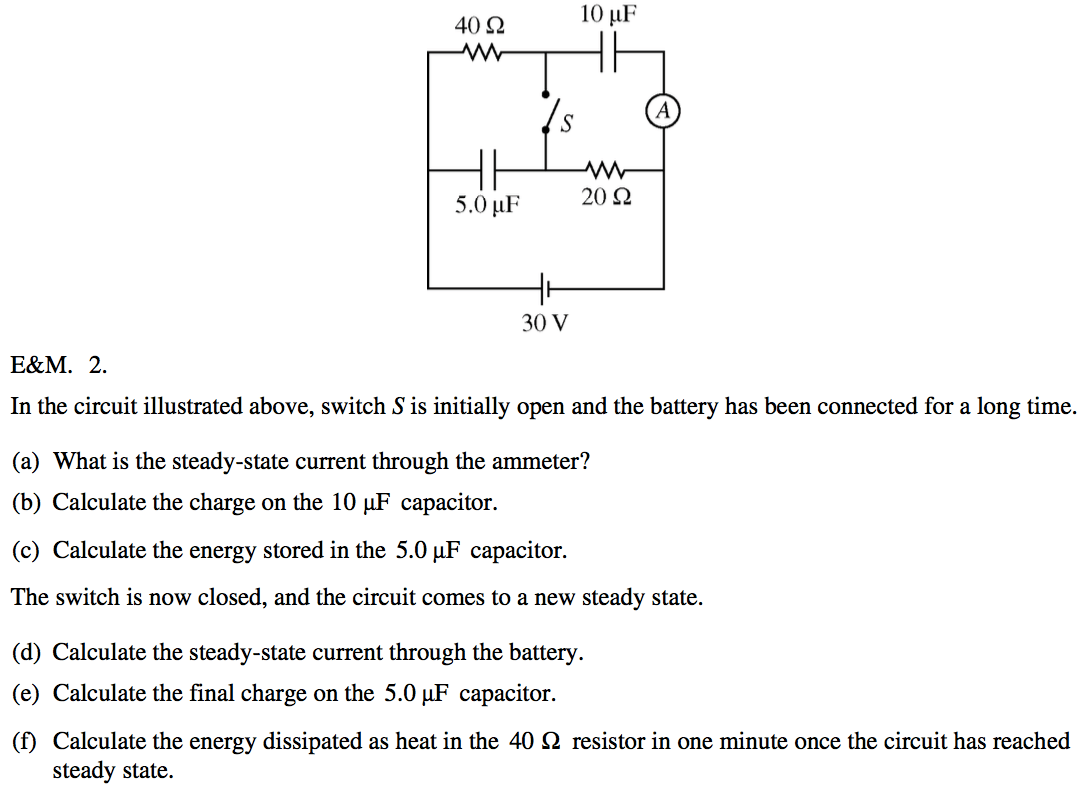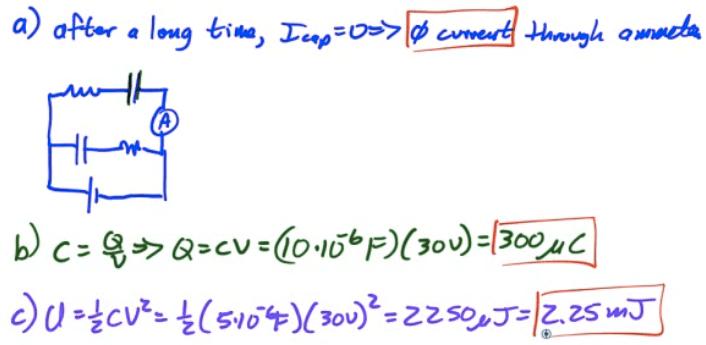# 2004 Free Response Question 2